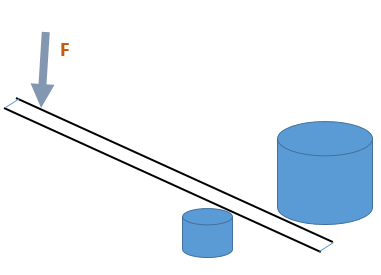# Introduction to Conservation of Energy

Conservation of energy indicates that the total energy (KE + PE) of a system stays the same.
Energy is conserved if there are no non-conservative forces doing work on the system.

Example 1:
Assume a lever is used to lift a box as shown in the figure below.Question: Do you think that the work done by the person on the lever be:
1. less than,
2. greater than,
3. or equal to the work done by the lever on the rock?
Answer: The work done by the person can never be less than the work done by the lever on the rock.
Assuming no dissipative forces they will be the same.
This example illustrates the conservation of energy.

Example 2:
In another example showing work done in pulling a sled up a hill generating an increase in PE of the sled and rider.
The initial energy is converted to KE as they slide down the hill. Any work done by frictional forces is considered negative W = −Fd.
That work removes mechanical energy from the system (see below):